© 2021 - First Crazy Developer (Abhishek Kumar)

###Importance of Generics in C#

In previous article we discussed about Collection in C#. After this article I felt that we also need to start discussion about "Generics". Now move a step ahead with discussion and today we will start discussion one of the most important chapter in C#.

But first, here’s an important question: "What is the most important point consider when we start coding in C#"?

Any guess.......

Here with cross finger I have two important points:

a.) Increased Code Reusability
b.) Type Safety

Do you have?
Answer is yes, you are good coder & no then you need to go through coding standard and practice.

Move ahead and start about Generics in C#. Generics provide the solution to a limitation in earlier versions of the common language runtime and the C# language in which generalization is accomplished by casting types to and from the universal base type Object. By creating a Generic Class, we can create a Collection that is type-safe at compile-time.

In order to understand the importance of this concepts and why they exist, again this article we'll need to hop into our time machine and take a look at some code from .NET 1.1.

First, we create a new console application with the name "GenericsSample" under in .Net 1.1 Framework and understand the limitations of using non-generic collection classes.

Look at the following code:

1. using System;
2. using System.Collections;
3. using System.Text;
4. namespace GenericsSample
5. {
6. class Program
7. {
8. static void Main(string[] args)
9. {
10. // The .NET Framework 1.1 way to create a list:
11. ArrayList intList = new ArrayList();
12. intList.Add(7);
13. intList.Add(16);
14. ArrayList stringList = new ArrayList();
15. stringList.Add("Dummy list first.");
16. stringList.Add("Dummy list second.");
17. }
18. }
19. }

In the above code we can see there's are variables named "intList & stringList". After declaration of variables we stored value into that. Here we used two variables to store integer and string value seprate. But we have an important question here- "What happen when the above code executed and what are the limitation of the above code"?

Any data types which added into an ArrayList is implicitly upcast to Object. If the items are value types, they must be boxed when they are added to the list, and unboxed when they are retrieved. Both the casting and the boxing and unboxing operations decrease performance; the effect of boxing and unboxing can be very significant in scenarios where you must iterate over large Collections. Here if we look the above code carefully get one more limitation, did you get that?

Again check the above code we get lack of compile-time type checking here; because an ArrayList casts everything to Object, there is no way at compile-time to prevent check this.

Look at the following code:

1. using System;
2. using System.Collections;
3. using System.Text;
4. namespace GenericsSample
5. {
6. class Program
7. {
8. static void Main(string[] args)
9. {
10. // The .NET Framework 1.1 way to create a list:
11. ArrayList intList = new ArrayList();
12. intList.Add(7);
13. intList.Add("Dummy list first.");
14. intList.Add(16);
15. intList.Add("Dummy list second.");
16. int a = 0;
17. // Error throw at run time when string value set in integer.
18. //But we don't get any error at compile time
19. foreach (int temp in intList)
20. {
21. a = a + temp;
22. }
23. }
24. }
25. }

When we compiled the above code that will be compiled successfully.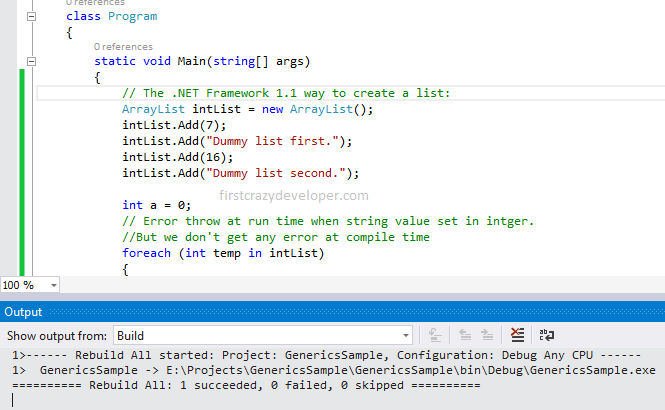After went through the above code and discussion we get that what ArrayList and other similar classes really need is a way for client code to specify, on a per-instance basis, the particular data type that they intend to use. That would eliminate the need for the upcast to T:System.Object and would also make it possible for the compiler to do type checking. In other words, here ArrayList needs a type parameter. That is exactly what Generics provide us in our code.

Now we move ahead and start coding with .Net Framework 2.0 where Microsoft introduce Collection with Generics and made the developer life easy. In the Generic List<T> Collection, in the N:System.Collections.Generic namespace, the same operation of adding items to the Collection resembles this.

Look at the following image and code: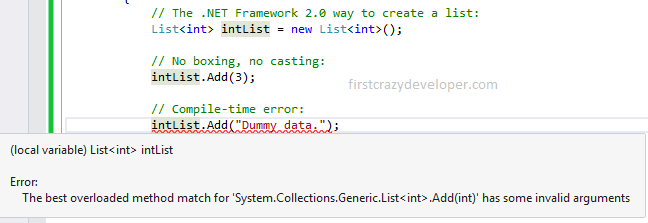1. using System;
2. using System.Collections.Generic;
3. using System.Text;
4. namespace GenericsSample
5. {
6. class Program
7. {
8. static void Main(string[] args)
9. {
10. // The .NET Framework 2.0 way to create a list:
11. List<int> intList = new List<int>();
12. // No boxing, no casting:
13. intList.Add(3);
14. // Compile-time error:
15. //intList.Add("Dummy data.");
16. }
17. }
18. }

In the above code & image we can see after Generics we don't need Boxing and Casting. We get compile time error when we try to set different data type. The most important point here we can just pass the data type in <> and List will work with that with type safe. This is an advantage of Generic with Collections. We can check every Collection work with Generics.

Look at the following code:

1. using System;
2. using System.Collections;
3. using System.Collections.Generic;
4. using System.Text;
5. namespace GenericsSample
6. {
7. class Program
8. {
9. static void Main(string[] args)
10. {
11. // The .NET Framework 2.0 way to create a different types of list:
12. List<int> intList = new List<int>();
13. Stack<int> intStack = new Stack<int>();
14. Queue<int> intQueue = new Queue<int>();
15. Dictionary<int, string> intDic = new Dictionary<int, string> ();
16. SortedList<int, string> intSortedList = new SortedList<int, string>();
17. SortedDictionary<int, string> intSortedDic = new SortedDictionary<int, string>();
18. }
19. }
20. }

In the above code we can see we have Generics facility with all Collection. These are not only safer than ArrayList, but also significantly faster, especially when the list items are value types.

After all the above discussion now we are in the position where we can say that "Generics permit classes, structs, interfaces, delegates, and methods to be parameterized by the types of data they store and manipulate."

We can find the more details about Generics terminology here: https://msdn.microsoft.com/en-us/library/ms172192.aspx

Now move ahead with an important question- "What are the benefits of Generic in C#?"

Look at the following code:

1. namespace GenericsSample
2. {
3. class Program
4. {
5. static void Main(string[] args)
6. {
7. List<float> listFloat = new List<float>();
8. List<string> listString = new List<string>();
9. List<mySample> listmySample = new List<mySample>();
10. List<myStructSample> listmyStructSample = new List<myStructSample>();
11. }
12. }
13. public class mySample
14. {
15. int temp = 0;
16. }
17. public struct myStructSample
18. {
19. int temp = 0;
20. }
21. }

In the above code we can see main benefit of Generics where in each of these instances of List<T>, every occurrence of T in the class will be substituted at run time with the type argument. By means of this substitution, we have created three separate type-safe and efficient objects using a single class definition.

Generics with User defined Class:

Look at the following image: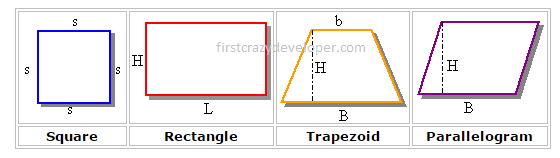We can see in the above image these are geometric figures with each having four sides. Now on the base of following image we can create a generic class. We can set a data type as an unknown type, anticipating that the dimensions of the figure can be considered as integer or double-precision types. Here is an example:

1. using System;
2. using System.Collections;
3. using System.Collections.Generic;
4. using System.Text;
5. namespace GenericsSample
6. {
7. class Program
8. {
9. static void Main(string[] args)
10. {
11. // Trapezoid with equal sides
12. var Kite = new GeometryDrawing<double>("Fly Kite", 18.64, 18.64);
13. Kite.ShowCharacteristics();
14. Console.WriteLine();
15. // Rectangle, in meters
16. var Stadium = new GeometryDrawing<int>();
17. Stadium.Name = "Dummy Stadium";
18. Stadium.Base = 15;
19. Stadium.Height = 28;
20. Stadium.ShowCharacteristics();
21. Console.WriteLine();
22. }
23. }
24. public class GeometryDrawing<T>
25. {
26. public virtual T Base {get;set;}
27. public virtual T Height {get;set;}
28. public virtual string Name { get; set; }
29. public GeometryDrawing (string name = "GeometryDrawing")
30. {
31. Name = name;
32. }
33. public GeometryDrawing(T bs, T height)
34. {
35. Name = "GeometryDrawing";
36. Base = bs;
37. Height = height;
38. }
39. public GeometryDrawing(string name, T bs, T height)
40. {
41. Name = name;
42. Base = bs;
43. Height = height;
44. }
45. public virtual string Describe()
46. {
47. return "A GeometryDrawing figure with four sides";
48. }
49. public virtual void ShowCharacteristics()
50. {
51. Console.WriteLine("Geometric Figure: {0}", Name);
52. Console.WriteLine("Description: {0}", Describe());
53. Console.WriteLine("Base: {0}", Base);
54. Console.WriteLine("Height: {0}", Height);
55. }
56. }
57. }

In the above code we can see there is Generic Class "GeometryDrawing" with three generic properties "Name, Base, Height". We used a virtual properties and method so this class can be use as base class in future. Most of place we used generic type place of fixed data types so our code can use with multiple data type. When we look into main method in first line we created an object name "Kite" as GeometryDrawing class type and here we are passing "double" data type. In further line we created one more object name "Stadium" as GeometryDrawing class type and here we are passing "int" data type.  Here we can see single Class use with two different data type.

This is the main advantage of Generic.

When we executed the above code we get following output: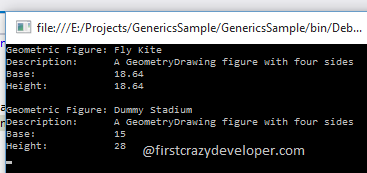Generic with Inheritance:

After the above code we extend that code to understand the concepts of Inheritance with Generic Class. Here now we make the above class as base class and create one more class.

Look at the following code:

1. public class Square<T> : GeometryDrawing<T>
2. {
3. public Square()
4. {
5. Name = "Square";
6. }
7. public Square(string name)
8. {
9. Name = "Square";
10. }
11. public Square (T side)
12. {
13. Name = "Square";
14. Base = side;
15. Height = side;
16. }
17. public Square(string name, T side)
18. {
19. Name = name;
20. Base = side;
21. Height = side;
22. }
23. public override string Describe()
24. {
25. return "A square is a geometric picture with four equal sides";
26. }
27. public override void ShowCharacteristics()
28. {
29. Console.WriteLine("Geometric Figure: {0}", Name);
30. Console.WriteLine("Description: {0}", Describe());
31. Console.WriteLine(" {0}", Describe());
32. Console.WriteLine("Side: {0}", Base);
33. }
34. }
35. // Rectangle, in meters
36. var footballground = new Square<Byte>();
37. footballground.Name = "Plate";
38. footballground.Base = 15;
39. footballground.Height = 28;
40. footballground.ShowCharacteristics();
41. Console.ReadLine();

In the above code we can see there is a Generic Class "Square" which inherited from base class "GeometryDrawing". Then we change the logic of base class for inherited class.

Generic Constraints: Parameter to a Class Type:-

Look at the following code:

1. public class Drawing<T> where T : class
2. {
3. }

In the above code we can see that we used Generic with constraints. In the above code we set class type constraint. When we try to pass value type as generic we will get compile time error.

Look at the following image: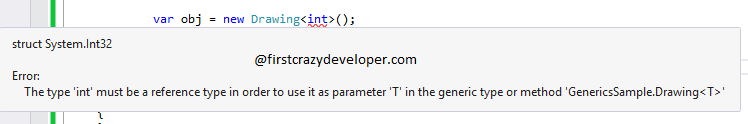In the above image we can see when we tried to pass value type we got compile error due to constraint use with the above class.

Generic Constraints: Parameter to a specific Class Type:-

Look at the following code:

1. public class Teacher<T>where T : Base
2. {
3. }

In the above code we can see that we used Generic with constraints. In the above code we set specific class type constraint. When we try to pass different class as generic we will get compile time error.

Look at the following image:In the above image we can see when we pass "Base" Class is working fine but when we tried to pass other Class we get compile time error.

Generic Constraints: Parameter to a new Default:-

Look at the following code:

1. public class Base : IBase
2. {
3. public virtual string FullName { get; set; }
4. public DateTime DateofBirth { get; set; }
5. // add default construtor
6. public Base()
7. {
8. }
9. public Base(string name)
10. {
11. FullName = name;
12. DateofBirth = new DateTime(0);
13. }
14. }
15. public class Student<T> where T : new()
16. {
17. public T StudentInfo { get; set; }
18. // add default construtor
19. public Student()
20. {
21. }
22. public Student(T record)
23. {
24. StudentInfo = record;
25. }
26. }

Now we use Generic in our main method:

1. Student<Base> student = new Student<Base>();

In the above code we can see there is added default constructor in both Base and Student Class. Now the above code working fine with new Default Constraints. Here we have an important question: "What will be happened if we remove the default constructor"?

Look at the following image:In the above image we can see when we tried to use this Generic type getting following compile type error- "must be a non-abstract type with a public parameter less constructor in order to use it as parameter 'T' in the generic type or method".

Generic with more than one parameter:

Look at the following code:

1. public interface IBase
2. {
3. int RollNumber { get; set; }
4. string FullName { get; set; }
5. DateTime DateofBirth { get; set; }
6. }
7. public class Base : IBase
8. {
9. public virtual string FullName { get; set; }
10. public virtual int RollNumber { get; set; }
11. public DateTime DateofBirth { get; set; }
12. // add default construtor
13. public Base()
14. {
15. }
16. public Base(string name, int rollnumber)
17. {
18. FullName = name;
19. DateofBirth = new DateTime(0);
20. RollNumber = rollnumber;
21. }
22. }
23. public class Category
24. {
25. public string Type{get;set;}
26. }
27. public class Student<T,P>
28. where T : Base
29. where P : Category
30. {
31. public T StudentInfo { get; set; }
32. public P StudentCategory { get; set; }
33. // add default construtor
34. public Student()
35. {
36. }
37. public Student(T studentinfo,P studentcategory)
38. {
39. StudentInfo = studentinfo;
40. StudentCategory = studentcategory;
41. }
42. }

In main method we use following code:

1. public interface IBase
2. {
3. int RollNumber { get; set; }
4. string FullName { get; set; }
5. DateTime DateofBirth { get; set; }
6. }
7. public class Base : IBase
8. {
9. public virtual string FullName { get; set; }
10. public virtual int RollNumber { get; set; }
11. public DateTime DateofBirth { get; set; }
12. // add default construtor
13. public Base()
14. {
15. }
16. public Base(string name, int rollnumber)
17. {
18. FullName = name;
19. DateofBirth = new DateTime(0);
20. RollNumber = rollnumber;
21. }
22. }
23. public class Category
24. {
25. public string Type{get;set;}
26. }
27. public class Student<T,P>
28. where T : Base
29. where P : Category
30. {
31. public T StudentInfo { get; set; }
32. public P StudentCategory { get; set; }
33. // add default construtor
34. public Student()
35. {
36. }
37. public Student(T studentinfo,P studentcategory)
38. {
39. StudentInfo = studentinfo;
40. StudentCategory = studentcategory;
41. }
42. }

In the above code we used Generic with multiple parameter "Base and Category" class. When we executed the above code got following output:After all of the above discussion I hope we can understand the concepts of Generics. There are lots of place where we can use Generics in our code and program. Look at the following point where we can use this concept:

1. Generic Type Parameters
2. Constraints on Type Parameters
3. Generic Classes
4. Generic Interfaces
5. Generic Methods
6. Generics and Arrays
7. Generic Delegates
8. default Keyword
9. Generic Extension

Happy reading!

Abhishek KumarHome Page 28 August 2015